# fx-9860GII SD

Close

• The colors may differ slightly from the original.

## fx-9860GII SD

SD Card Slot
AP / SAT / SAT Subject / PSAT / NMSQT / ACT / IB
Suitable for AP, SAT, SAT Subject, PSAT, NMSQT, ACT & IB examinations.
Number of Functions : Over 2900
Natural textbook display
Display expression same as textbook.

## Features### User-friendly Interface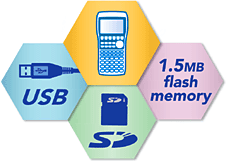• Rectangular coordinate graphing, Polar coordinate graphing
• Integration graph
• Parametric function graphing, Inequality graphing
• Trace, Zoom (box zoom, zoom in, zoom out, auto zoom)
• Table and Graph
• Dual Graph (table and graph, graph and graph)
• Sketch (tangent line, normal line, inverse function)
• Solve (root, minimum, maximum, intersection, integration)
• Dynamic graph
• Conic section graph
• Recursion graph
• List-based one-variable and two-variable statistical analysis
• Statistical regression calculations
• Statistical plot (scatter plot, xyLine, normal probability plot, histogram, box plot)
• Statistical regression graphs (linear, med-med, quadratic, cubic, quartic, logarithmic, exponential, power, sinusoidal, logistic regression)
• Advanced statistical calculations: tests (Z-test, t-test, Chi square test, F-test, ANOVA), intervals (Z-interval, t-interval), distributions
• Pie chart
• Bar graph
• Power functions (square root, cubic root, square, power, radical root)
• GCD/LCM
• Coordinate conversion (Pol, Rec)
• Combination/Permutation (nCr, nPr)
• Factorial, Inverse, random numbers, Fractions
• Logical operations
• Matrix calculations
• Complex number calculations
• Base- n calculations/conversions
• List data calculations
• Metric Conversion
• Natural format equation output
• Calculation history
• Numeric equation solver, simultaneous equations, polynomial equations
• Financial calculations
• Programming
• SD memory card slot (fx-9860GII SD only)
• Data communication
• User memory: 62,000 bytes, User Storage memory: 1.5 M bytes

### Hardware Features

#### High-resolution LCD

The large 64 × 128-dot display of the fx-9860GII Series high-resolution LCD produces formulas, graphs and graphics that are sharper, clearer, and easier to read.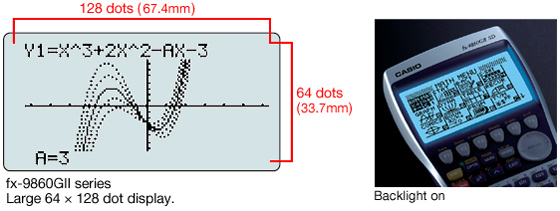#### High-speed CPU

A high-performance, high-speed CPU gives fx-9860GII Series calculators processing speeds that are three to five time faster than other brand calculators in their class. Processes and plots encountered in complex calculations and graphics are handled with ease, for enhanced operational efficiency and learning as well.

#### Out-of-the-box USB Operations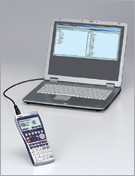A USB cable, unit-to-unit cable and Program-Link Software all are included with the calculator, so high-speed data communication with a computer as well as unit-to-unit data and program transfers can be performed virtually out of the box.

#### SD Memory Card Slot (SD model only)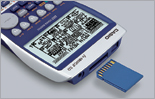The fx-9860GII SD is equipped with an SD memory card slot for easy data transfers.

### Natural textbook display!

CASIO's original "Natural Expression Input Display" and "Natural Expression Output Display" make it possible to display fractions, exponents, logarithms, powers, and square roots just as they are written in the textbook. The result is enhanced student comprehension and improved math class efficiency.

#### Natural Input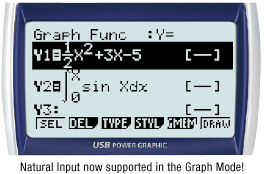#### Natural Output

Polynomial equation result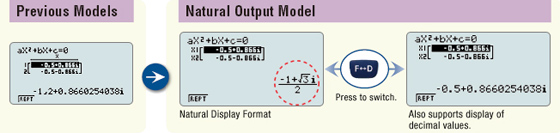Result of expression with Pi symbol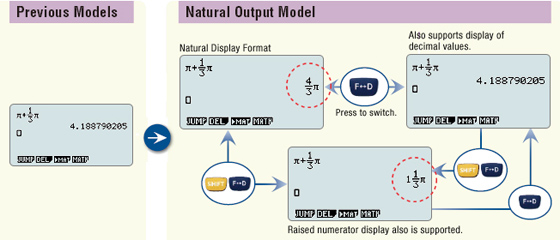### eActivity

fx-9860GII Series calculators come with the same eActivity capabilities that originally appeared on the ClassPad 330. Now teachers as well as students can create their own problems and study materials. Students get the opportunity to learn at their own pace for more efficient study both at school and at home. eActivity is a great motivator for learning and understanding.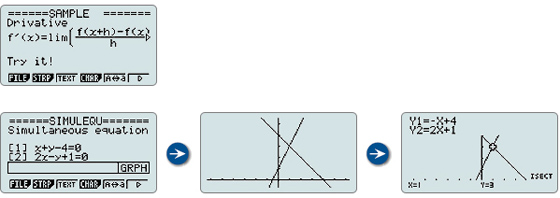### Built-in Software

A multi-function spreadsheet with built-in graphing capabilities is a valuable tool for table calculation lesson exercises.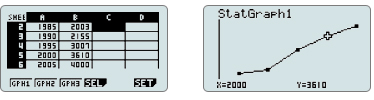#### E-CON2

E-CON2 provides total control over the optional EA-200 Data Analyzer. It makes it possible to measure changes in temperature, sound, or speed using the EA-200 without any troublesome settings or program input.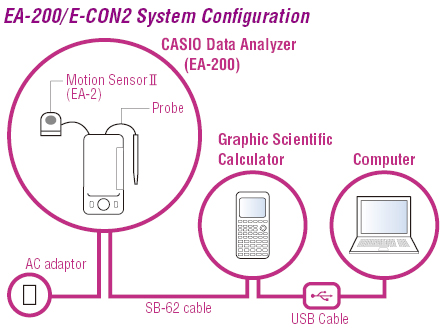#### Pre-installed Software

Pre-installed add-in software comes installed on the calculator when you purchase it. You can use such software as-is, or you can delete it to free up memory.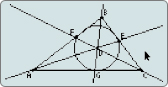Geometry

Geometry add-in software is designed to make learning geometry fun.

Physium

The Physium add-in provides instant access to the periodic table of elements, whose data can be used in calculations. Often-use elements and atomic symbols can be stored for quick and easy recall whenever you need them.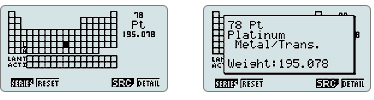### Other Features

#### Probability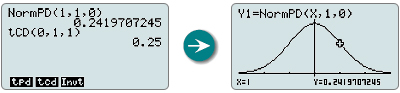Normal distribution, student's t-distribution, and other often-used statistical calculations are provided in function format for easier practical application.

#### Inequality Graphing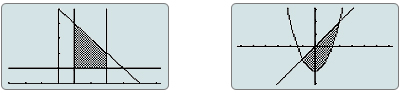New support for graphing the inequality of an x=Constant graph and x=f ( y) graph allows study of the area for which the x-range is defined.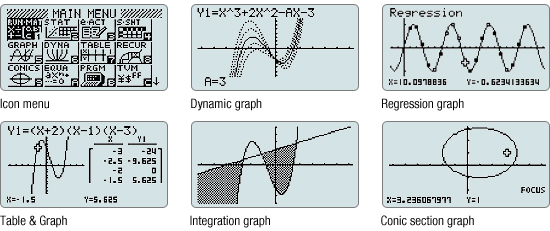#### List-based Statistics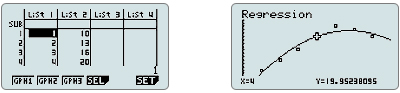Store a list of values in memory for use when performing function and statistical calculations, when drawing graphs, or when generating tables of numeric values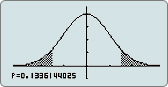Perform tests, confidence interval, probability distribution, and other calculations and graphing.

1-sample t-test graph

#### Financial Calculations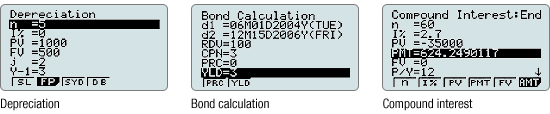• Depreciation
• Bond calculation
• Simple interest
• Compound interest
• Investment appraisal (cash flow)
• Amortization
• Interest rate conversion (annual percentage rate and effective interest rate)
• Cost, selling price, or margin
• Day or date calculations

#### Peripherals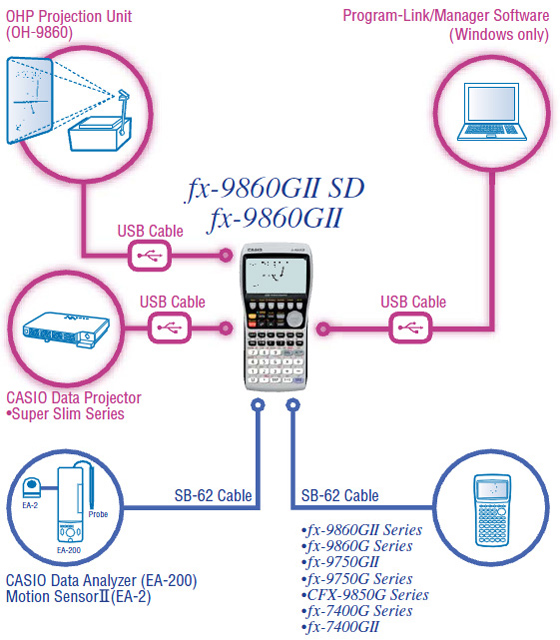## Specifications

• Programmable

•SD Card Slot

•AP / SAT / SAT Subject / PSAT / NMSQT / ACT / IB Suitable for AP, SAT, SAT Subject, PSAT, NMSQT, ACT & IB examinations.

•Number of Functions : Over 2900

•Natural textbook display Display expression same as textbook.

•10 + 2 digits 10-digit mantissa + 2-digit exponential display.

•Dot matrix display High-resolution screen provides beautiful looking graphs every time.

•Icon menu Specify the operation you want to perform by selecting an icon or inputting a number.

•Multi-replay Quick and easy recall of previously executed formulas for editing and re-execution.

•Plastic keys Designed and engineered for easy operation.

•List based STAT-data editor Viewing and editing of input data in list format, showing data groups (x-data, y-data, frequency) and surrounding data.

• Basic Statistics Standard statistics functions such as Mean, SUM, Standard Deviation, and Regression

• Basic Mathematical Functions Trigonometric, Exponential logarithmic, etc.

• Table Generation You can create a numerical table based on that expression by registering a function expression. In addition, graph functions can be represented by graphs.

• Data Logger You can obtain data log of scientific experiments.

• Intermediate Statistics More statistical functions such as Paired-variable statistical calculation, Quartiles and List display functions for speedy and thorough learning .

• Scientific Constants You can use constant symbols used in physics, science classes.

• Financial Financial functions are built in, making financial calculations easy.

• Graphing

• Vector

• Advanced Statistics Support high level statistics by 7 kinds of advanced distribution calculation.

•Data communication with a personal computer Allows data communication with a personal computer.

• AAA-size Battery

• Differentiation

• Connection to PC

• Matrix

• Equation

• Integration

•Metric conversion function You can convert a number in one unit to a number in a different unit.

• Complex number calculation

• High Resolution We are choosing a model with higher definition in our non-programmable model and a higher definition model with Programmable model.